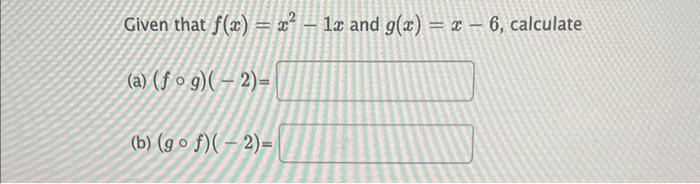Home / Expert Answers / Algebra / given-that-f-x-x-2-1-x-and-g-x-x-6-calculate-a-f-circ-g-2-b-g-pa560

# (Solved): Given that \( f(x)=x^{2}-1 x \) and \( g(x)=x-6 \), calculate (a) \( (f \circ g)(-2)= \) (b) \( (g ...Given that \( f(x)=x^{2}-1 x \) and \( g(x)=x-6 \), calculate (a) \( (f \circ g)(-2)= \) (b) \( (g \circ f)(-2)= \)

We have an Answer from Expert